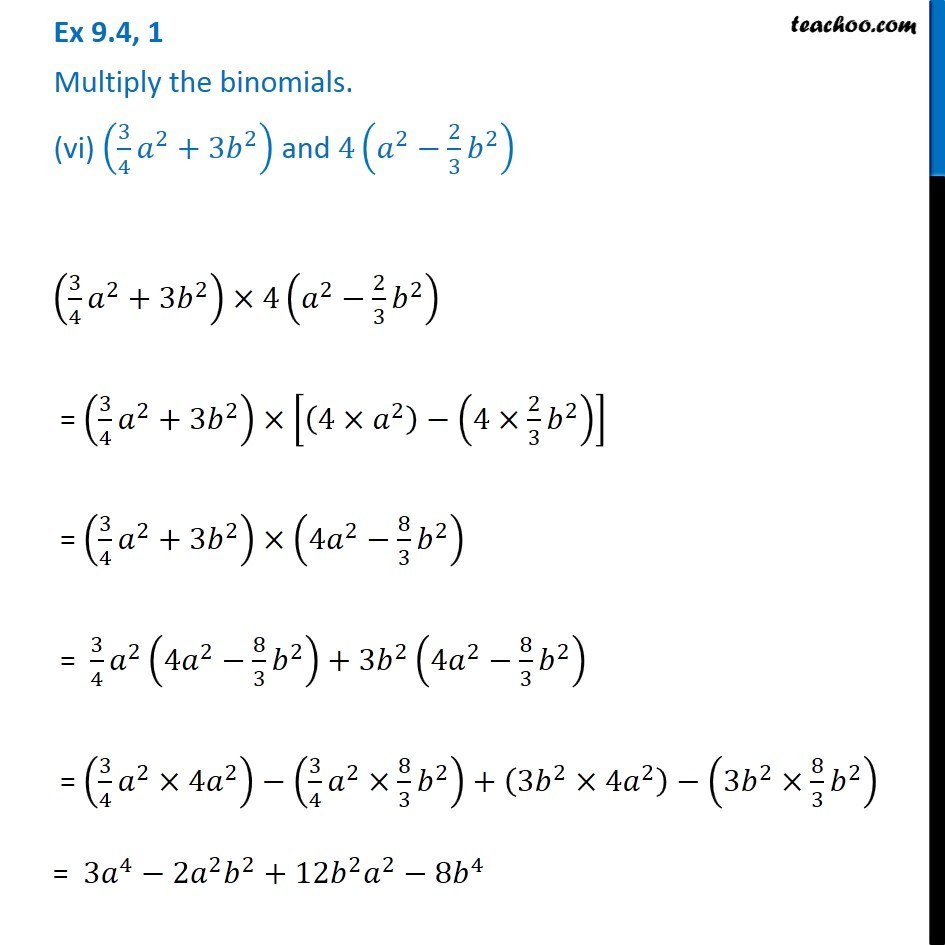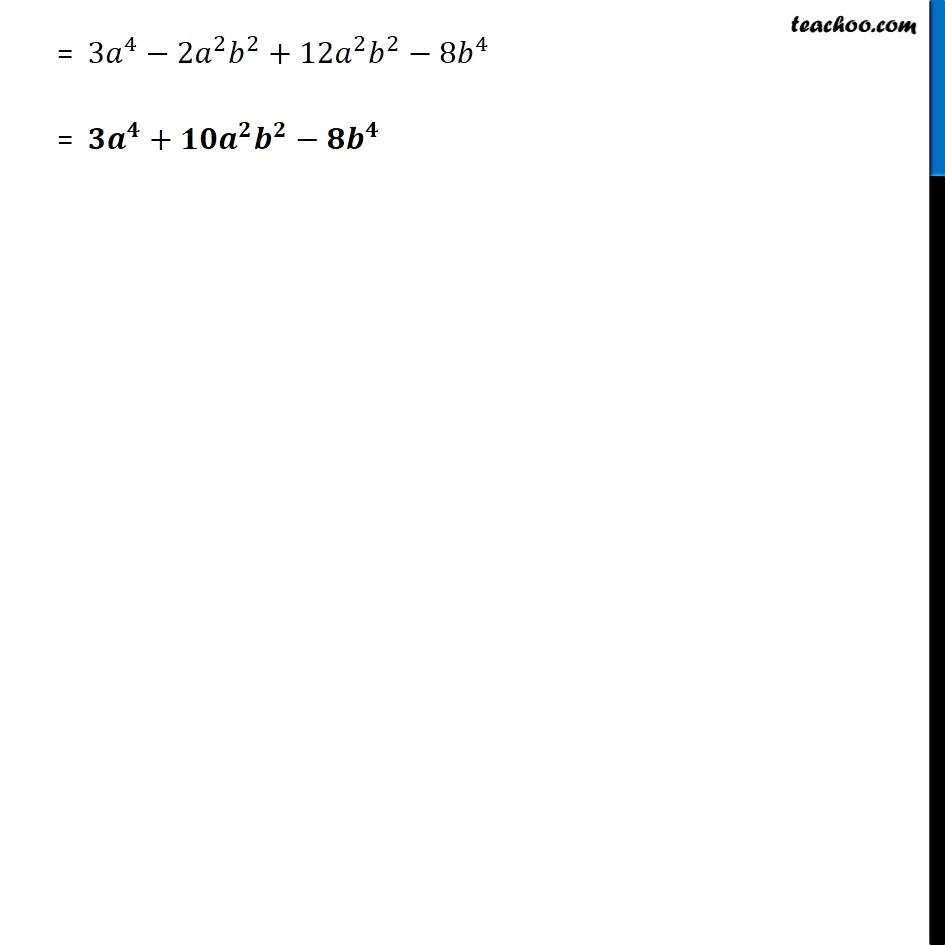Subscribe to our Youtube Channel - https://you.tube/teachoo

1. Chapter 9 Class 8 Algebraic Expressions and Identities
2. Concept wise
3. Multiplication of Polynoimals by Polynomials

Transcript

Ex 9.4, 1 Multiply the binomials. (vi) (3/4 𝑎^2+3𝑏^2 ) and 4(𝑎^2−2/3 𝑏^2 ) (3/4 𝑎^2+3𝑏^2 )×4(𝑎^2−2/3 𝑏^2 ) = (3/4 𝑎^2+3𝑏^2 )×[(4×𝑎^2 )−(4×2/3 𝑏^2 )] = (3/4 𝑎^2+3𝑏^2 )×(4𝑎^2−8/3 𝑏^2 ) = 3/4 𝑎^2 (4𝑎^2−8/3 𝑏^2 )+3𝑏^2 (4𝑎^2−8/3 𝑏^2 ) = (3/4 𝑎^2×4𝑎^2 )−(3/4 𝑎^2×8/3 𝑏^2 )+(3𝑏^2×4𝑎^2 )−(3𝑏^2×8/3 𝑏^2 ) = 3𝑎^4−2𝑎^2 𝑏^2+12𝑏^2 𝑎^2−8𝑏^4

Multiplication of Polynoimals by Polynomials

About the AuthorDavneet Singh
Davneet Singh is a graduate from Indian Institute of Technology, Kanpur. He has been teaching from the past 9 years. He provides courses for Maths and Science at Teachoo.